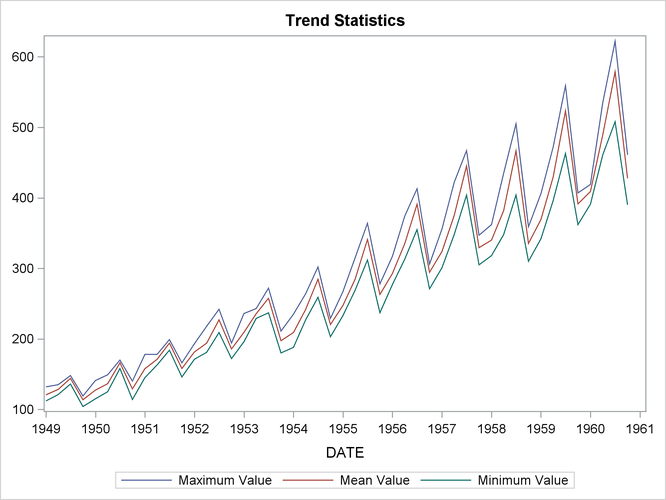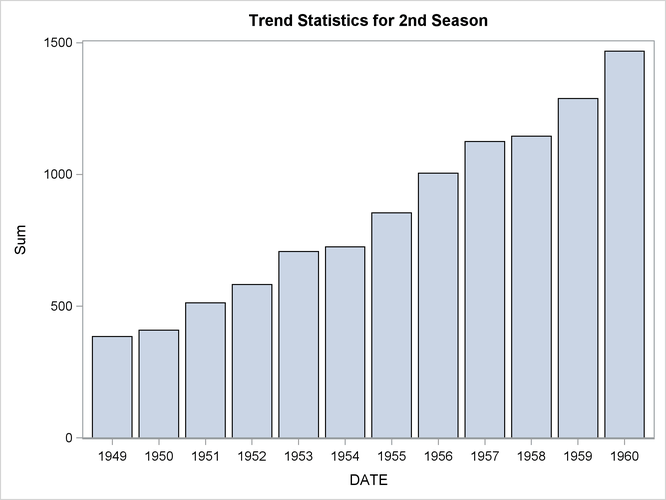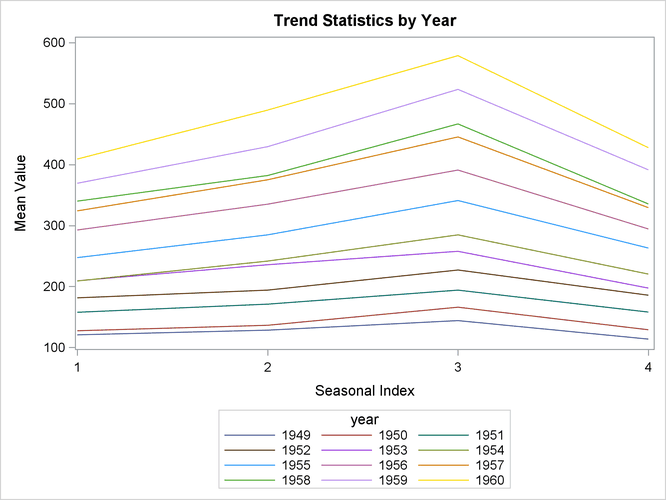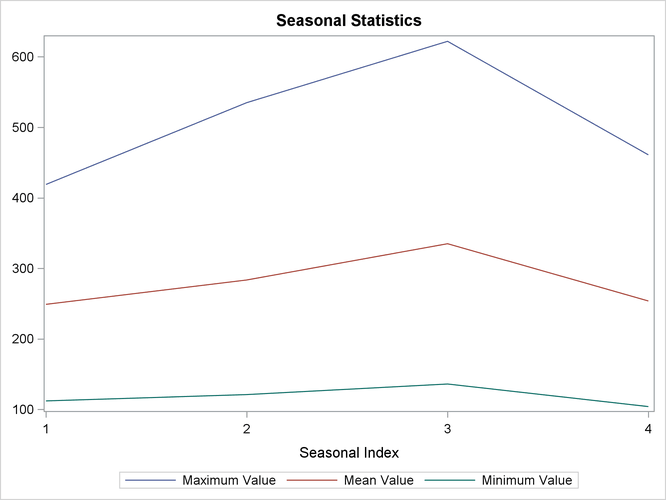# The TIMESERIES Procedure

### Example 32.2 Trend and Seasonal Analysis

This example illustrates using the TIMESERIES procedure for trend and seasonal analysis of time-stamped transactional data.

Suppose that the data set `SASHELP.AIR` contains two variables: `DATE` and `AIR`. The variable `DATE` contains sorted SAS date values recorded at no particular frequency. The variable `AIR` contains the transaction values to be analyzed.

The following statements accumulate the transactional data on an average basis to form a quarterly time series and perform trend and seasonal analysis on the transactions.

```proc timeseries data=sashelp.air
out=series
outtrend=trend
outseason=season print=seasons;
id date interval=qtr accumulate=avg;
var air;
run;
```

The time series is stored in the data set `WORK.SERIES,` the trend statistics are stored in the data set `WORK.TREND,` and the seasonal statistics are stored in the data set `WORK.SEASON.` Additionally, the seasonal statistics are printed (PRINT=SEASONS) and the results of the seasonal analysis are shown in Output 32.2.1.

Output 32.2.1: Seasonal Statistics Table

The TIMESERIES Procedure

Season Statistics for Variable AIR
Season Index N Minimum Maximum Sum Mean Standard Deviation
1 36 112.0000 419.0000 8963.00 248.9722 95.65189
2 36 121.0000 535.0000 10207.00 283.5278 117.61839
3 36 136.0000 622.0000 12058.00 334.9444 143.97935
4 36 104.0000 461.0000 9135.00 253.7500 101.34732

Using the trend statistics stored in the `WORK.TREND` data set, the following statements plot various trend statistics associated with each time period over time.

```title1 "Trend Statistics";
proc sgplot data=trend;
series x=date y=max  / lineattrs=(pattern=solid);
series x=date y=mean / lineattrs=(pattern=solid);
series x=date y=min  / lineattrs=(pattern=solid);
yaxis display=(nolabel);
format date year4.;
run;
```

The results of this trend analysis are shown in Output 32.2.2.

Output 32.2.2: Trend Statistics PlotUsing the trend statistics stored in the `WORK.TREND` data set, the following statements chart the sum of the transactions associated with each time period for the second season over time.

```title1 "Trend Statistics for 2nd Season";
proc sgplot data=trend;
where _season_ = 2;
vbar date / freq=sum;
format date year4.;
yaxis label='Sum';
run;
```

The results of this trend analysis are shown in Output 32.2.3.

Output 32.2.3: Trend Statistics Bar ChartUsing the trend statistics stored in the `WORK.TREND` data set, the following statements plot the mean of the transactions associated with each time period by each year over time.

```data trend;
set trend;
year = year(date);
run;

title1 "Trend Statistics by Year";
proc sgplot data=trend;
series x=_season_ y=mean / group=year lineattrs=(pattern=solid);
xaxis values=(1 to 4 by 1);
run;
```

The results of this trend analysis are shown in Output 32.2.4.

Output 32.2.4: Trend StatisticsUsing the season statistics stored in the `WORK.SEASON` data set, the following statements plot various season statistics for each season.

```title1 "Seasonal Statistics";
proc sgplot data=season;
series x=_season_ y=max  / lineattrs=(pattern=solid);
series x=_season_ y=mean / lineattrs=(pattern=solid);
series x=_season_ y=min  / lineattrs=(pattern=solid);
yaxis display=(nolabel);
xaxis values=(1 to 4 by 1);
run;
```

The results of this seasonal analysis are shown in Output 32.2.5.

Output 32.2.5: Seasonal Statistics Plot